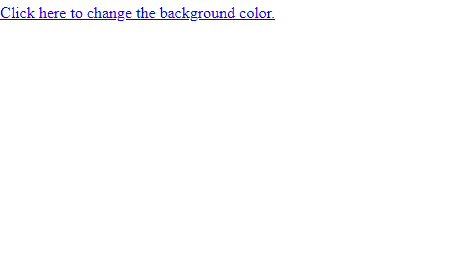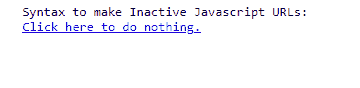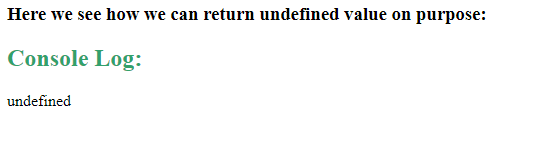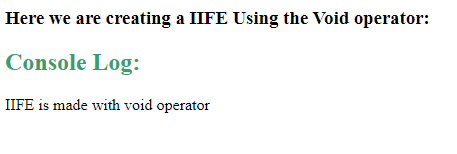# What is void and when to use void type in JavaScript ?

• Last Updated : 31 Dec, 2021

In this article, we will know what is void operator in Javascript, along with discussing the particular condition where void operator can be used & understanding their implementation through the examples.

The void keyword in JavaScript, is used to evaluate an expression which does not return any value. The void operator is an unary operator that accepts the single operand, which may be of any type. The importance of the void keyword come into role when we just need to evaluate an expression instead of returning its value. It means, by using it, we can prevent the browser from displaying the result of the execution of the expression.

Syntax:

```void expression
void(expression)```

The void operator has an operator precedence ie., the priority will be given to operators while parsing a statement that has more than one operator performing operations in it. Here, we have used the parenthesis, to express the purpose of the expression, according to its precedence.

For instance, consider the below example:

```void (10 == '10') // undefined
void 10 == '10' // false```

For the 1st case, when the number is compared with string, inside the parenthesis with the void keyword, it returns undefined whereas in the 2nd case, when the expression is evaluates directly with the void keyword, it returns false.

Example: This example describes the returning undefined value.

## HTML

 `<``script``>``       ``function foo() {``           ``return void 0;``       ``}``       ``console.log(foo());```

Output:

`undefined`

void 0 can be used as a placeholder URL that represents an onclick event is tied to the link to perform the actual action. The void 0 is also commonly used in code downsizing, as it is a way of writing undefined.

There are 3 instances where the void operator can be used:

Active Javascript URLs: The javascript: is referred to as the pseudo URL, when we provide it as a value of “href” in an anchor tag, the browser evaluates the expression that follows the “:” symbol. On the other hand, the expression that follows the “:” is usually used as a referenced path.

The void operator is most commonly used in managing the javascript: URL (s) because it allows the browser to show the end result of the evaluation of the expression rather than the returned value of the evaluated expression on the client side.

Example: In this example, the link changes the color of the background to green without returning any value to the browser.

## HTML

 ```<``html``>`` ` `<``head``>``    ``<``title``>Using Void in URLs```` ` `<``body``>``    ``<``a` `href``=``"javascript:void(document.body.style.backgroundColor='#32CD32');"``>``        ``Click here to change the background color.``    `` ```` ` ``

Output:Inactive Javascript URLs: In some cases, it is not required by a link to navigate anywhere or do anything. To achieve it, we combine the pseudo URL (javascript:) with void(0) as a value of an href, it tells the browser to return/do nothing when that link is clicked.

Example: This example explains the generating the inactive Javascript URL.

## HTML

 `<``html``>`` ` `<``head``>``      ``<``title``>Creating Inactive URL````   ` `<``body``>``    ``<``pre``>``        ``Syntax to make Inactive Javascript URLs:``        ``<``a` `href``=``"javascript:void(0)"``>Click here to do nothing.``    `` ```` ` ``

Output: The link will be recognized by the browser but the link doesn’t react at all. When 0 is passed to void as an argument, it does nothing or returns nothing. Here “Click here and do nothing” link doesn’t do anything at all, as depicted in the output.Suppression of Arrow Functions: Arrow functions provide a braceless syntax to return the value of an expression. To ensure that the return value of the function expression (when it is of no use) doesn’t affect the code in any way, it can be passed into a void operator.

Example: This example explains the returning the value of an expression.

## HTML

 `<``html``>`` ` `<``head``>``    ``<``title``>Returning the value of an expression```` ` `<``body``>``    ``<``h3``>Here we see how we can return undefined value on purpose:``     ` `    ``<``script``>``        ``function someOtherFunction(num) {``            ``return num + 1``        ``}``        ``const toReturnUndefined = () => void someOtherFunction(1);``        ``console.log(toReturnUndefined());``    `````` ` ``

Output:Returning Undefined Value on Purpose: We can convert any variable’s value to an undefined type.

## HTML

 `<``html``>`` ` `<``head``>``    ``<``title``>Returning Undefined Value on Purpose```` ` `<``body``>``    ``<``h3``>Click the following to Return the Undefined Value:``    ``<``input` `type``=``"button"` `           ``value``=``"Click Me"` `           ``onclick``=``"genUValue();"` `/>``     ` `    ``<``script``>``        ``function genUValue() {``            ``var g, f, h;``            ``g = void(f = 13, h = 19);``            ``document.write('g = ' + g);``        ``}``    `````` ` ``

Output:Immediately Invoked Function Expression: An Immediately Invoked Function Expression(IIFE) is a type of Javascript function which gets executed as soon as it gets defined. They are very useful because they don’t affect the global object and the remote variable declarations very easily. We can use the void operator to create IIFEs. It will force the function to be treated as an expression rather than a declaration.

Example:

## HTML

 `<``html``>`` ` `<``head``>``    ``<``title``>Creating a IIFE Using the Void operator```` ` `<``body``>``    ``<``h3``>Here we are creating a IIFE Using the Void operator:``     ` `  ``<``script``>``        ``void function iife() {``            ``console.log("IIFE is made with void operator");``        ``}();``        ``iife();``  `````` ` ``

Output:My Personal Notes arrow_drop_up